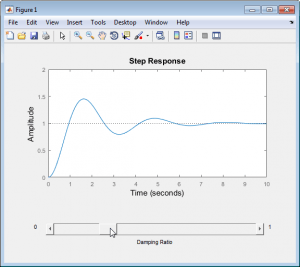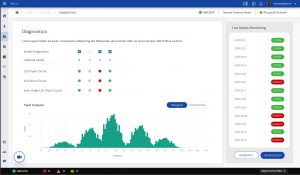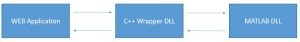# Transforming MATLAB Evaluation GUI to Modern Web-GUI

MATLAB as you all know is a popular programming language that is extensively used for computation expensive solutions. MATLAB is best suited for developing image processing algorithms, signal processing algorithms, and GUI (Graphical User Interface) applications as it has readily available libraries that help in faster development. MATLAB natively supports hardware interface (like connecting with Evaluation Modules (EVM)) and it has rich inbuilt plot functions that help in visualizing data as graphs easier which in turn makes it a go-to product for developing Evaluation GUI.

Evaluation GUI is similar to other normal GUIs but these GUIs are mostly used in the evaluation of semiconductor chips. These GUIs have features centered around visualizing and benchmarking the performance of the chips. Though MATLAB has numerous features, it suffers from the following drawbacks when distributing MATLAB solution as an installer to end-customer:

1. The UI (User Interface) and UX (User Experience) of the GUI (Graphical User Interface) developed using MATLAB are generally difficult to follow

2. Since MATLAB is computationally expensive, complex GUI applications built on MATLAB will tend to be slow

To overcome this, we can use web-based applications to develop GUI and MATLAB can be used to process the required data. Web Applications are similar to the normal application except that it runs on a web browser instead of the host OS. Web applications can be accessed by anyone with or without an internet connection depending on the application’s architecture and intended use-case. So why web-based application?

1. Most of the technologies used in web-technology are completely open-source e., we can edit and redistribute applications built using these technologies free of cost.

2. There are numerous open-source frameworks and packages available like Angular, bootstrap which can be used to make the look and feel of the GUI better

3. Rich documentation and large active developer base

4. The application can be distributed as a standalone GUI application or link to a webpage which can be opened anywhere using the Internet

5. Let’s visually compare the GUI developed using MATLAB and Web-Technology

MATLAB GUI:Web-GUI:It can be seen that the UI and UX of Web GUI is more appealing than MATLAB

How to Integrate MATLAB with web-based GUI applications:

There are multiple ways to Integrate MATLAB with GUI applications such as:

1. Running MATLAB exe and opening a port for communication through which both the application can exchange information

2. Converting MATLAB into DLLs and creating a C++ wrapper to call these MATLAB DLLs.

3. Converting MATLAB into C, C++ DLLs (it seems possible with newer versions)

Among the above three possible solutions, I will be explaining the second solution.

DLLs (Dynamic Linked Libraries) can be used to establish communication between two different software. It contains functions, classes, variables, and resources (such as icons, images, files, etc.) that an EXE or other DLL uses. In Windows, these files are represented as .dll and .so in Linux/Unix environment.

MATLAB natively supports converting the MATLAB source code into DLLs which can be used in other applications. Web-based GUIs are generally built using HTML, CSS, and JavaScript (JS). Node.js is an environment that runs JavaScript code and NPM (Node Package Manager) is a package manager for JavaScript packages. It contains numerous JavaScript packages that are open-sourced and help in developing solutions faster.

JavaScript does not natively support linking MATLAB DLLs but it is possible to link C++ DLL. There are many NPM packages that can be used for linking DLLs and “node-ffi” is one such package. To link MATLAB DLLs to a web-based applications, a C++ wrapper needs to be created. This wrapper can be used to interact with MATLAB functions in the DLL. This wrapper can be converted into DLLs and can be linked to the web-based application. Diagrammatically the whole process can be represented as:There are numerous open-source graph tools available for web applications that can be used to visualize data more intuitively and user friendly. Combining these we can create a GUI with improved UI/UX design, process the data using MATLAB functions, and visualize the processed data as a graph.

The graphs developed can be more intuitive in UI perspective as well as on the user experience. These graphs play a key role in evaluating a chip’s performance with help of EVM boards in the semiconductor industry.

A better Interface will always have an upper hand in attracting customers.

Thus, it is possible to replace a MATLAB GUI with a web-based application that can be distributed to end-customers.

One thing to be noted is that the MCR (MATLAB Compiler Runtime) needs to be installed in the target PC (i.e.) the PC where the web-based application runs. MCR is important to run MATLAB DLLs. Though MATLAB is proprietary software, MCR can be installed free of cost.